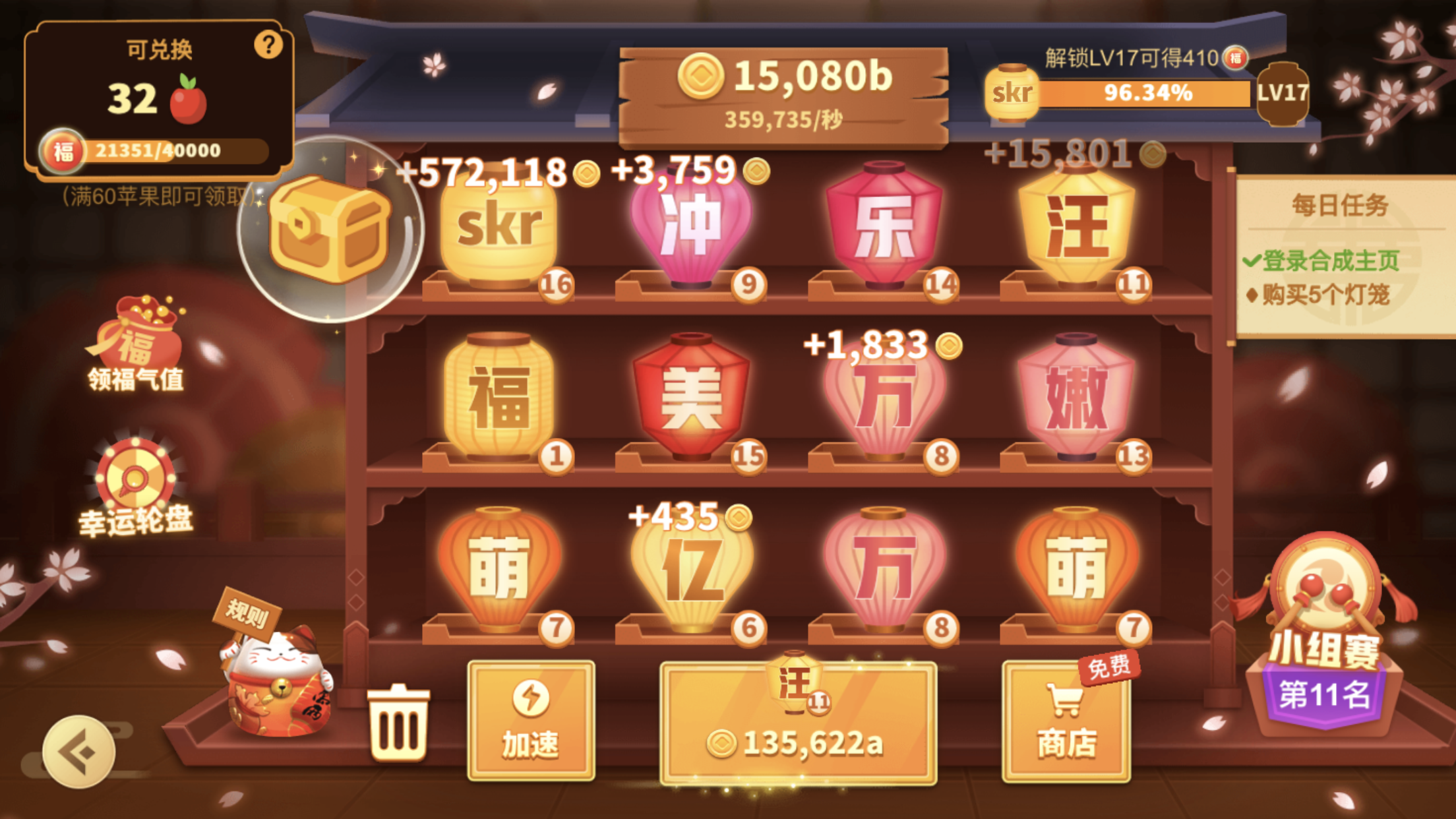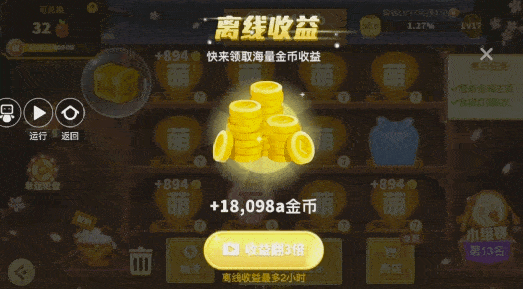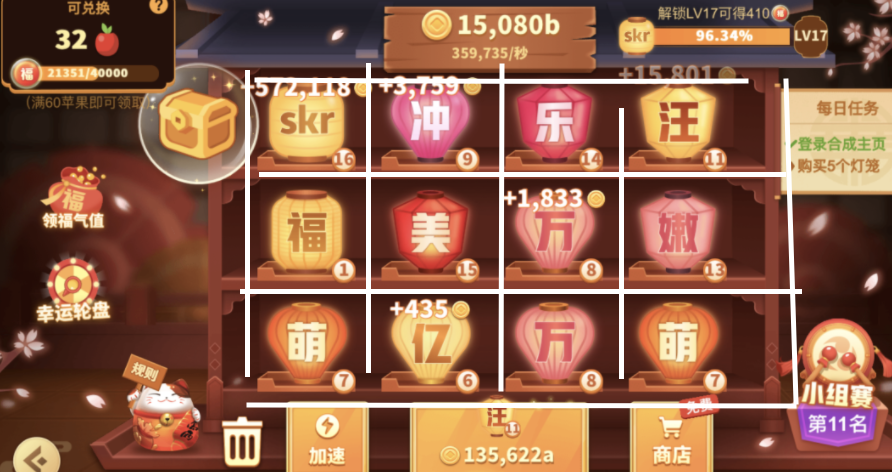# 2020年 IOS 逆向 反编译 注入修改游戏或APP调用参数系列新手教程——按键精灵脚本来模拟合成灯笼

@[TOC](2020年 IOS 逆向 反编译 注入修改游戏或APP调用参数系列新手教程——按键精灵脚本来模拟合成灯笼)

# 开篇

2020年 IOS 逆向 反编译 注入修改游戏或APP调用参数系列新手教程，之所有特别提出是2020年的是因为很多老的方法失效了，网络上大部分的教程都是基于2018年左右的IOS10及以下老版本来■■，而且教程新手很难看明白，当当就安装这些■■工具的环境的坑之多就足以劝退很多新手。本系列教程会列出■■的环境及工具版本，尽量避免新手入坑。

# 需求&最终效果# 进入正题

## 思路## 实现代码

``````ShowMessage "启动脚本。。。。。"

// 横屏
//x
//|__ y

Dim offsetX = 260
Dim offsetY = 292

Dim CloseXPoint = array(988,2010)
// 3 个特征点 array(y, x)
Dim point1 = array(876, 760)
Dim point2 = array(833,777)
Dim point3 = array(894,757)
// 第一个灯笼的特征
Dim firstDengLong = array(array(point1(0), point1(1), GetPixelColor(point1(0), point1(1))), array(point2(0), point2(1), GetPixelColor(point2(0), point2(1))), array(point3(0), point3(1), GetPixelColor(point3(0), point3(1))) )

Dim dengLongArr = array()

Dim i=0,count=0
// 所有灯笼的比较坐标点，12个 [[[5,3],[3,1]] , [[5,3],[3,1]]]
// 12 次循环
For i = 0 To 2
dim j = 0
For j = 0 To 3
dengLongArr(count) = array()
// 类似 dengLongArr[count]
dengLongArr(count, 0) = Array(firstDengLong(0, 0) - i * offsetX, firstDengLong(0, 1) + j * offsetY)
dengLongArr(count, 1) = Array(firstDengLong(1, 0) - i * offsetX, firstDengLong(1, 1) + j * offsetY)
dengLongArr(count, 2) = Array(firstDengLong(2, 0) - i * offsetX, firstDengLong(2, 1) + j * offsetY)
count = count + 1
Next
Next

// 合并灯笼
Function heBingDengLong()
i = 0
j = 0
// 第一个灯笼的特征
Dim firstDengLong = array(array(point1(0), point1(1), GetPixelColor(point1(0), point1(1))), array(point2(0), point2(1), GetPixelColor(point2(0), point2(1))), array(point3(0), point3(1), GetPixelColor(point3(0), point3(1))) )
Dim currDengLongColorArr = array()
// 11 次循环
For i = 0 To 11

currDengLongColorArr = array( GetPixelColor(dengLongArr(i,0,0), dengLongArr(i,0,1)), GetPixelColor(dengLongArr(i,1,0), dengLongArr(i,1,1)), GetPixelColor(dengLongArr(i,2,0), dengLongArr(i,2,1)))

For j = 1 To 11
closeX ()
closeComm()
TracePrint "这一点"
dim str = dengLongArr(j,0,0)&"|"& dengLongArr(j,0,1) & "|" & currDengLongColorArr(0) & "-101010," & dengLongArr(j,1,0)&"|"& dengLongArr(j,1,1) & "|" & currDengLongColorArr(1) & "-101010," & dengLongArr(j,2,0)&"|"& dengLongArr(j,2,1) & "|" & currDengLongColorArr(2) & "-101010"

If Not(i = j) and CmpColorEx(str, 0.9 ) = 1 Then
TracePrint 	 str & "找到没有" & i+1 & "->" & j+1
// 合并灯笼
heBingDengLongTouch (dengLongArr(i,0,0), dengLongArr(i,0,1), dengLongArr(j,0,0), dengLongArr(j,0,1))
//EndScript
//Exit For
Else
//TracePrint 0

End If
Next

Next

End Function

Function hebingDengLong2()
i = 0
j = 0
Dim temp
// 11 次循环
For i = 0 To 10
closeX ()
closeComm()
temp = i
For j = 0 To 10
heBingDengLongTouch (dengLongArr(temp,0,0), dengLongArr(temp,0,1), dengLongArr(j,0,0), dengLongArr(j,0,1))
temp = j
Next

Next
End Function

// 移动指定灯笼到第一位置
Function moveToFirst(number)
TracePrint "----------------------------" & number
TouchDown dengLongArr(number-1,0,0),dengLongArr(number-1,0,1), 1//按住屏幕上的100,100坐标不放，并设置此触点ID=1
TouchMove firstDengLong(0,0),firstDengLong(0,1), 1//将ID=2的触点花200毫秒移动至500,500坐标
TouchUp 1//松开弹起ID=1的触点
// TracePrint "移动力"
End Function

// 拖拽合并
Function heBingDengLongTouch(x1, y1, x2, y2)
TracePrint "----------------------------"
TouchDown x1,y1, 1//按住屏幕上的100,100坐标不放，并设置此触点ID=1
TouchMove x2, y2, 1//将ID=1的触点花200毫秒移动至500,500坐标
TouchUp 1//松开弹起ID=1的触点

End Function

// 关
Function closeX()
// 免费升级的点击
//各种弹层的关闭处理
End Function

Function closeComm()
// 也是各种弹层的关闭处理
End Function

Function isInGameWindow()
// 是否在游戏窗口的判断
End Function

// 广告处理
// 各种广告处理。。。。
TracePrint "开始广告处理................"
// 存在倒计时形式的x按钮

// 误入横版的app store 显示页面
End Function

// 脚本主函数
Function heBingDengLongMain()
For ii = 1 To 12
closeX ()
closeComm()
// 原始的判断逻辑，效率低
hebingDengLong()
// 如果想用第二种合并处理逻辑的话，上面的For可以去掉，下面的moveToFirst()也要去掉
//hebingDengLong2()
moveToFirst (ii)
Else
TracePrint "不合并"
End If
Next

End Function

If isInGameWindow() = False Then
TracePrint "不在游戏界面"
ShowMessage "不在游戏界面"
Delay 2000
Else
heBingDengLongMain()
End If

``````

Dim point1 = array(876, 760)
Dim point2 = array(833,777)
Dim point3 = array(894,757)

Dim offsetX = 260
Dim offsetY = 292

firstDengLong是指第一灯笼的特征。

isInGameWindow（）是判断当前窗口是否在游戏界面，先讲是的情况，会进入到heBingDengLongMain（）就是合并灯笼的主主函数。首先执行closeX ()和 closeComm()是为了关闭游戏界面的离线收益遮罩层，因为经常会弹出翻倍和升级提示的窗口，不关闭触摸就失效了。总之人的操作是什么顺序逻辑的，脚本也是根据各种情况来做判断的。然后进入具体合并逻辑hebingDengLong（），通过数组的遍历，逐个拿出来和第一个灯笼的特征比较，如果特征一样证明是相同级别的灯笼可以合并，

moveToFirst (ii)的操作，就是保证每个灯笼有机会移动至第一个格子，有被拿来比较的机会。

# 额外

## shell 定时任务

linux的定时任务是通过crontab来配置的，而ios是通过LaunchDaemons来实现的。配置文件写在/Library/LaunchDaemons/下面。

``````<?xml version="1.0" encoding="UTF-8"?>
<!DOCTYPE plist PUBLIC "-//Apple//DTD PLIST 1.0//EN" "http://www.apple.com/DTDs/PropertyList-1.0.dtd">
<plist version="1.0">
<dict>
<key>Label</key>
<string>com.apple.mycrontest.plist</string>
<key>ProgramArguments</key>
<array>
<string>/bin/bash</string>
<string>/var/root/myshelltest.sh</string>
</array>
<key>StartInterval</key>
<integer>1</integer>
</dict>
</plist>

``````

``````launchctl load /Library/LaunchDaemons/com.apple.mycrontest.plist
``````

``````launchctl unload /Library/LaunchDaemons/com.apple.mycrontest.plist
``````

# 博文主索引目录入口

2020年 IOS 逆向 反编译 注入修改游戏或APP的调用参数新手系列教程主目录入口

7 个赞